eric chapman

•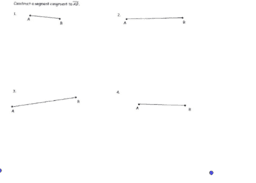Geometric Constructions

Activity

eric chapman

•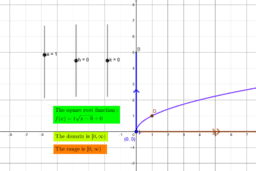Property of square root graph

Activity

eric chapman

•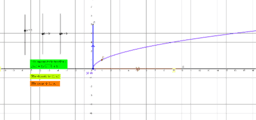Property of square root graph

Activity

eric chapman

•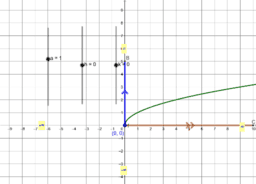Property of square root graph

Activity

eric chapman

•Assignment 8.1

Activity

eric chapman

•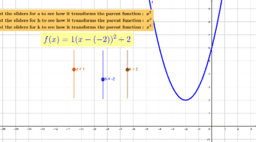Vertex form of a quadratic tool

Activity

eric chapman

•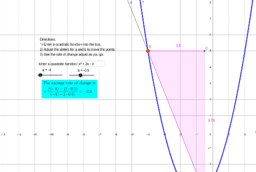Average Rate of Change tool

Activity

eric chapman

•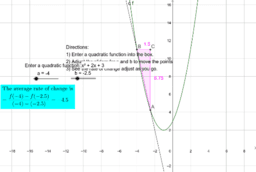Average Rate of Change tool

Activity

eric chapman

•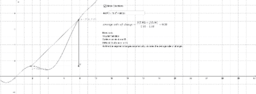Copy of Average Rate Of Change

Activity

eric chapman

•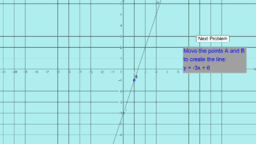graph a line

Activity

eric chapman

•graph a line

Activity

eric chapman

•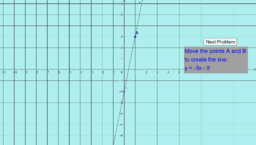Graph a line

Activity

eric chapman

•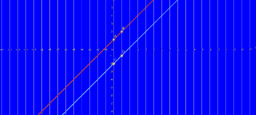Activity

eric chapman